## Wednesday, December 23, 2015

### DTT and the pattern of comparative data

A colleague recently inquired as to how one might go about interpreting the pattern shown by a so-called disparity-through-time (DTT) plot.

A DTT plot shows the mean disparity (effectively, variance) of each subtree compared to the total disparity, and is calculated by taking a time slice at each 'event' (speciation event recorded in the reconstructed phylogeny) of the tree.

DTT plots are interpreted in a variety of ways in the literature - for instance, as evidence that variability accumulates within, rather than between, clades; or that phenotypic diversity has tended to accrue in the early rather than the later stages of a diversification.

I thought it might be nonetheless useful to conduct a 'mini' simulation study in which I examined how, in general, the accumulation of disparity among clades through time may be related to biological process.

To start with, here are a couple of small custom functions that I wrote to facilitate the analyses:

``````meanDtt<-function(D){
max.t<-max(sapply(D,function(x) max(x\$times)))
t<-seq(0,1,by=0.01)*max.t
d<-vector()
for(i in 1:length(t)){
d[i]<-0
for(j in 1:length(D)){
ii<-max(which(D[[j]]\$times<=t[i]))
d[i]<-d[i]+D[[j]]\$dtt[ii]/length(D)
}
}
obj<-list(times=t,dtt=d)
obj
}
plotDtt<-function(D,main=""){
max.d<-max(sapply(D,function(x) max(x\$dtt)))
max.t<-max(sapply(D,function(x) max(x\$times)))
plot.new()
plot.window(xlim=c(0,max.t),ylim=c(0,max.d))
for(i in 1:length(D)) lines(D[[i]]\$times,D[[i]]\$dtt,
col=rgb(0,0,1,0.1),lwd=2,
type="s")
axis(1)
axis(2)
title(xlab="time",ylab="disparity",main=main)
obj<-meanDtt(D)
lines(c(0,obj\$times),c(1,obj\$dtt),lwd=2)
}
``````

Next, we can load our packages. DTT calculation is part of the geiger package.

``````library(phytools)
``````
``````## Loading required package: ape
##
##  # ATTENTION: maps v3.0 has an updated 'world' map.        #
##  # Many country borders and names have changed since 1990. #
##  # Type '?world' or 'news(package="maps")'. See README_v3. #
``````
``````library(geiger)
``````

OK, now we can simulate some trees:

``````trees<-pbtree(n=100,scale=1,nsim=200)
trees
``````
``````## 200 phylogenetic trees
``````

OK, now let's explore the relationship between process & DTT. Our custom function, `plotDtt`, plots all the DTTs but also overlays the average DTT across simulations.

First, Brownian motion:

``````## Brownian motion
X<-lapply(trees,fastBM)
D<-mapply(dtt,trees,X,MoreArgs=list(plot=FALSE),SIMPLIFY=FALSE)
plotDtt(D,main="Brownian motion")
``````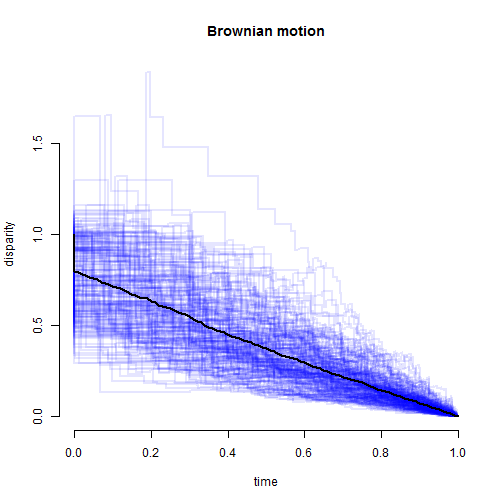Now, early-burst evolution:

``````## early burst
X<-lapply(trees,function(x) fastBM(rescale(x,"EB",a=-4)))
D<-mapply(dtt,trees,X,MoreArgs=list(plot=FALSE),SIMPLIFY=FALSE)
plotDtt(D,main="Early-Burst")
``````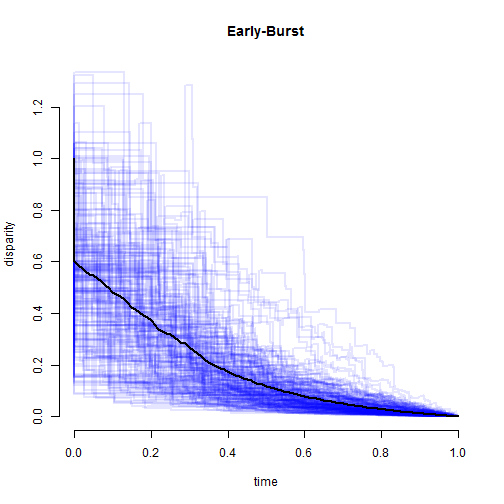Late-burst:

``````## late burst
X<-lapply(trees,function(x) fastBM(rescale(x,"EB",a=4)))
D<-mapply(dtt,trees,X,MoreArgs=list(plot=FALSE),SIMPLIFY=FALSE)
plotDtt(D,main="Late-Burst")
``````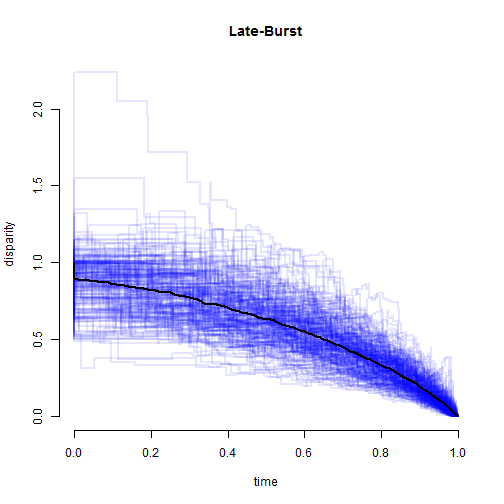Now, how about the Ornstein-Uhlenbeck model with a single optimum:

``````## OU
X<-lapply(trees,function(x) fastBM(rescale(x,"OU",alpha=4)))
D<-mapply(dtt,trees,X,MoreArgs=list(plot=FALSE),SIMPLIFY=FALSE)
plotDtt(D,main="Ornstein-Uhlenbeck")
``````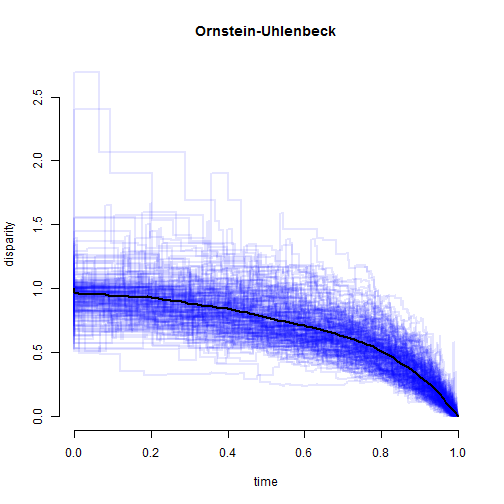In addition, here are a couple of models that we can simulate very easily, but not fit:

``````## Brownian motion with bounds
X<-lapply(trees,fastBM,bounds=c(-1,1))
D<-mapply(dtt,trees,X,MoreArgs=list(plot=FALSE),SIMPLIFY=FALSE)
plotDtt(D,main="Bounded BM [-1,1]")
``````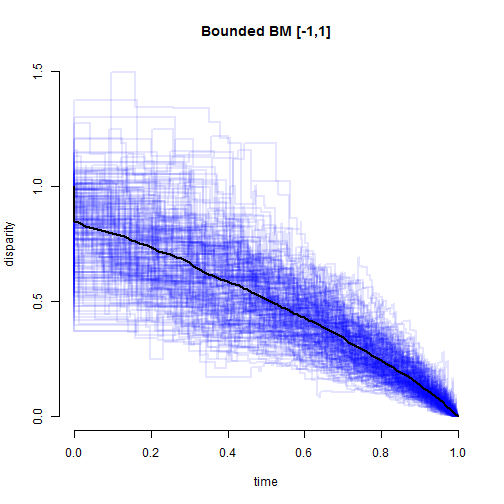``````X<-lapply(trees,fastBM,bounds=c(-0.4,0.4))
D<-mapply(dtt,trees,X,MoreArgs=list(plot=FALSE),SIMPLIFY=FALSE)
plotDtt(D,main="Bounded BM [-0.4,0.4]")
``````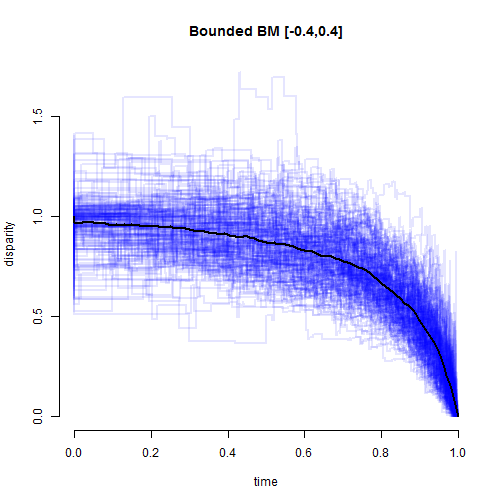``````X<-lapply(trees,fastBM,bounds=c(-0.2,0.2))
D<-mapply(dtt,trees,X,MoreArgs=list(plot=FALSE),SIMPLIFY=FALSE)
plotDtt(D,main="Bounded BM [-0.2,0.2]")
``````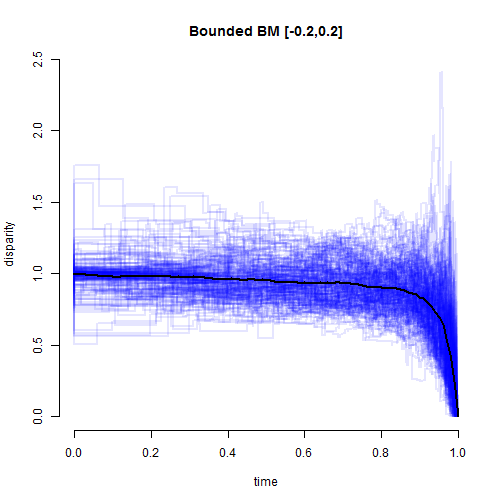``````## Brownian motion with a trend
X<-lapply(trees,fastBM,mu=4)
D<-mapply(dtt,trees,X,MoreArgs=list(plot=FALSE),SIMPLIFY=FALSE)
plotDtt(D,main="BM with a trend")
``````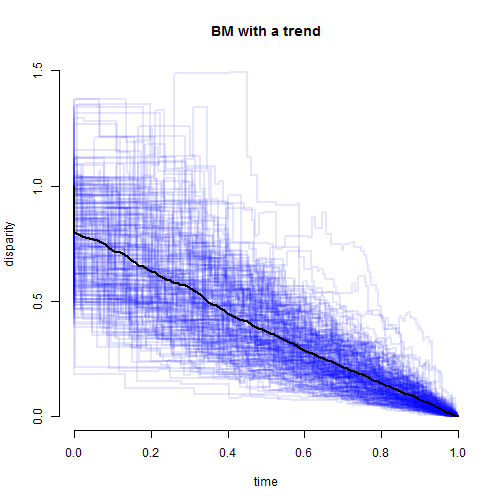Finally, it is important to keep in mine that DTT plots can be pretty substantially affected by sampling error in the estimation of species means. Here is a demo of the effect of two different levels of sampling error. I have simulated sampling variances from a exponential distribution with rate 1.0, but then multiplied by different values of a constant `k`, in which larger values imply more sampling error:

``````## measurement error
sim.error<-function(tree,k=0.1){
x<-fastBM(tree)
v<-k*setNames(rexp(n=Ntip(tree)),names(x))
sampleFrom(x,v,n=rep(1,Ntip(tree)))
}
X<-lapply(trees,sim.error,k=0.01)
D<-mapply(dtt,trees,X,MoreArgs=list(plot=FALSE),SIMPLIFY=FALSE)
plotDtt(D,"BM with sampling error (k=0.01)")
``````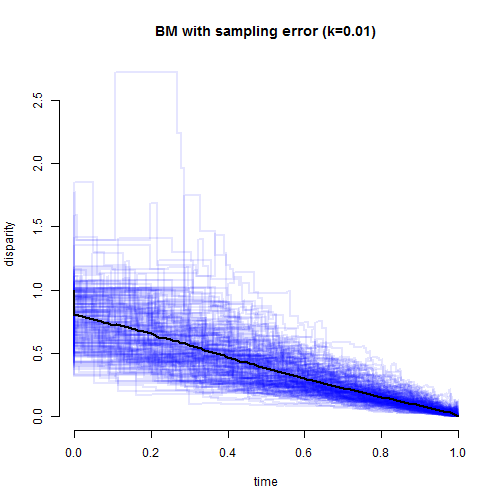``````X<-lapply(trees,sim.error,k=0.05)
D<-mapply(dtt,trees,X,MoreArgs=list(plot=FALSE),SIMPLIFY=FALSE)
plotDtt(D,"BM with sampling error (k=0.05)")
``````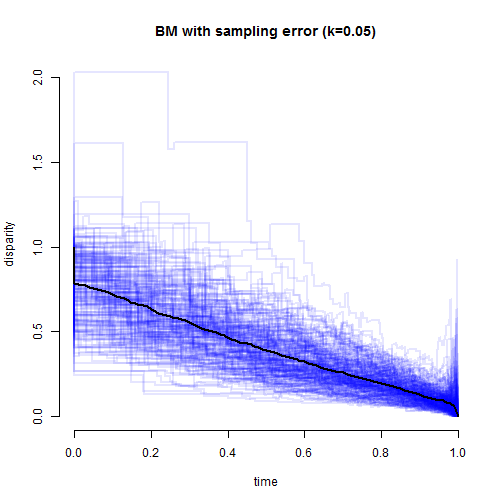``````X<-lapply(trees,sim.error,k=0.2)
D<-mapply(dtt,trees,X,MoreArgs=list(plot=FALSE),SIMPLIFY=FALSE)
plotDtt(D,"BM with sampling error (k=0.2)")
``````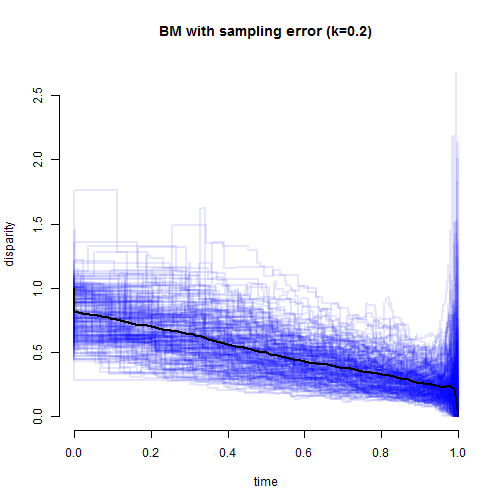That's really all I have.